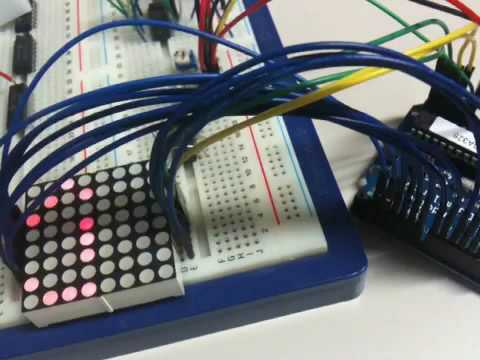# Arduino Row-column Scanning to control an 8×8 LED Matrix Code

LED displays are often packaged as matrixes of LEDs arranged in rows of common anodes and columns of common cathodes, or the reverse. Here’s a typical example, and its schematic:These can be very useful displays. To control a matrix, you connect both its rows and columns to your microcontroller. The columns are connected to the LEDs cathodes (see Figure 1), so a column needs to be high for any of the LEDs in that column to turn on. The rows are connected to the LEDs anodes, so the row needs to be low for an individual LED to turn on. If the row and the column are both high or both low, no voltage flows through the LED and it doesn’t turn on.

### Circuit

The 16 pins of the matrix are hooked up to 16 pins of the Arduino. Four of the analog pins are used as digital inputs 16 through 19. The order of the pins is assigned in two arrays in the code.

Two potentiometers, connected to analog pins 0 and 1, control the movement of a lit LED in the matrix.

image developed using Fritzing. For more circuit examples, see the Fritzing project page

Schematic:### Code

```/*
Row-Column Scanning an 8x8 LED matrix with X-Y input

created 27 May 2009
modified 30 Aug 2011
by Tom Igoe

This example works for the Lumex  LDM-24488NI Matrix. See
http://sigma.octopart.com/140413/datasheet/Lumex-LDM-24488NI.pdf
for the pin connections

For other LED cathode column matrixes, you should only need to change
the pin numbers in the row[] and column[] arrays

rows are the anodes
cols are the cathodes
---------

Pin numbers:
Matrix:
* Digital pins 2 through 13,
* analog pins 2 through 5 used as digital 16 through 19
Potentiometers:
* center pins are attached to analog pins 0 and 1, respectively
* side pins attached to +5V and ground, respectively.

This example code is in the public domain.

http://www.arduino.cc/en/Tutorial/RowColumnScanning

*/```

For more detail: Arduino Row-columm Scanning to control an 8×8 LED Matrix Code

#### This Post / Project can also be found using search terms:

• stp16cp05 led matrix arduino example
• Arduino 8X8 LED Matrix Code
• arduino button matrix
• arduino matrix projects and code